## Introduction

The proliferation of satellites in Earth orbit, which are increasing in both number and value, makes the problem of collisions with orbital debris very real. One of the most practical solutions to this problem is debris removal facilitated by a ground-based pulsed laser. In this approach, laser pulses ablate debris material, change the debris velocity and move the debris to a lower orbit, where natural burn-up occurs (Figure 1). This method of debris removal has been analyzed by the ‘Orion’ project;1,2 in this analysis, requirements for the laser and optical and tracking systems were summarized, and the role of nonlinear effects was discussed. Two aspects of the situation have changed since the completion of that project. First, the risk of valuable-asset damage has increased and is now so serious that governments may be willing to spend money on orbital-debris removal. Second, a significant advance in powerful pulsed-laser technology has taken place, mainly at Lawrence Livermore National Laboratory, with the completion of the National Ignition Facility Project.3 Systems designed for inertial-confinement-fusion applications are a near-perfect fit for orbital-debris-removal applications.

We begin the analysis with the requirements for the laser pulse on the target. Then, we discuss beam propagation and focusing to more completely define the requirements for the laser. Based on these more specific requirements, we specify a range of parameters for laser operation. We demonstrate that the laser-pulse power substantially exceeds the critical power for self-focusing in air. However, because the laser light is propagated almost vertically, the self-focusing length is much longer than the thickness of the atmosphere. Our numerical calculations demonstrate that the spatial structure of the beam on the target is smooth, without filaments, but the nonlinear effects noticeably decrease the peak intensity. We demonstrate that the atmosphere can be treated as an additional focusing lens and that preliminary beam defocusing can significantly compensate for the detrimental effects of the atmosphere.

The detrimental effects of nonlinearity can be greatly reduced if the laser is placed at a high elevation. This reduction is the result of the decreased air density and reduced the atmospheric thickness through which the beam propagates when the laser is at a high elevation.

In the last section, we discuss the role of additional nonlinear effects, including the beam broadening caused by atmospheric turbulence. We demonstrate that these detrimental effects are important, but we argue that proper optimization of the laser and beam-control system renders the ground-based laser space-debris cleaning approach feasible.

## Materials and methods

In this section, we formulate the required parameters for the laser system, including the laser-pulse characteristics, following previous research by Rubenchik et al.4 We start from the interaction of radiation with debris. High-intensity pulsed-laser radiation that is incident on debris vaporizes the surface material, creating recoil momentum that changes the debris velocity. It is clear that an optimal laser intensity exists for any specified pulse duration. At low intensity, the surface temperature and evaporation rate are low, and the recoil momentum is small. At high intensity, a large fraction of the laser energy is used to create a plasma, which contributes little to changing the momentum of the debris. A crucial parameter for pulsed-laser debris removal is the coupling coefficient Cm, which is the ratio of momentum imparted to the target to the incident laser energy, CmP/E. A review of data illustrating the dependence of Cm on intensity for various materials has been presented by Phipps.2,5 Experimental data from various groups demonstrate that for broad ranges of wavelength, pulse duration and pulse energy, the maximum coupling coefficient is reached at the intensity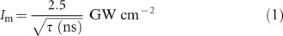where τ (ns) is the pulse duration. This numerical coefficient was determined for Al alloys, but does not change substantially for different materials and wavelengths. The temporal dependence indicates that the surface temperature and ablation are controlled by the thermal flux from the surface. As a function of laser intensity, Cm exhibits a peak that is not far from the vaporization threshold. At this threshold, plasma begins to be generated and absorptivity increases rapidly, which explains the weak sensitivity to the target material. Typical values of Cm are 1–10 dyne W−1.5 Below Im, the coupling coefficient drops sharply as the intensity decreases, while above Im, the coupling coefficient gradually decreases as the intensity increases. The fluence that corresponds to the optimal coupling is given by: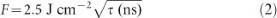We now derive the requirement for the laser-pulse energy that corresponds to delivering the optimal fluence to debris targets. The energy delivered by the laser to the vicinity of the target is required to be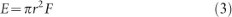where r is the radius of the beam in the target plane and F is the fluence. An approximate expression for the beam radius that accounts for beam quality and beam diffraction is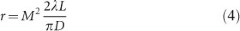where M2 is a factor that describes the beam quality with respect to an ideal Gaussian beam, λ is the laser wavelength, L is the path length from the beam director to the target and D is the diameter of the beam director. The effects of propagation through the atmosphere have, thus far, been ignored. The required laser-pulse energy E for delivering the pulse fluence required for optimal coupling is found by combining Equations (3)–(5), which yields: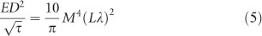We now consider a specific example, in which λ=1 µm, L=1000 km, D=2 m and M2=2, the latter of which is a value that can be achieved for high-energy lasers using spatial filters and adaptive optics systems. The path length L=1000 km is chosen to represent the altitude where the debris is most concentrated.2 For this case, r=64 cm, and the required pulse energy is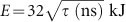. For a solid-state National Ignition Facility-like laser system of short pulse duration, the output energy is limited by nonlinear effects in the optical elements. For longer pulses, the energy is limited by the saturation of the extracted energy. The optimal pulse duration and energy for this type of laser are approximately 4 ns4 and E≈64 kJ, respectively.

For the above parameters, the laser power is 16 TW, which is well above the critical power for self-focusing in air: Pcr=4.3 GW for 1 µm light. Even for the ideal beam quality M2≈1, the required power exceeds 1000Pcr. Atmospheric turbulence and nonlinear effects can also further increase the required power. Clearly, the effects of nonlinearity on beam propagation must be considered.

## Results and discussion

We begin with a discussion of the modeling of nonlinear beam propagation. To avoid unnecessary complication, we first present the key concept using a simplified, although meaningful, model. The basic model reads as follows: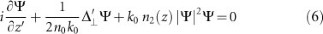Here, we consider a vertically propagating laser beam (compare to Ref. 6). This situation is not very different from the optimal angle for debris interaction, which is approximately 30° from the vertical.4 This simplification is not important, but it will simplify the presentation.

It is customary to introduce dimensionless variables: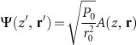where the dimensionless variables are z=z′/LD and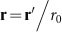; here,where k0=2π/λ0 and λ0=1.06 μm, k0=5.93 μm−1, n0=1.0 and n2(0)=4.2×10−19 cm2 W−1. Here, z=0 corresponds to sea level. We assume the commonly used exponential density dependence with an atmosphere height of 6 km: n(z)/n(0)=exp (−z/Z0), Z0=6 km. The nonlinear effects decay with height as n2(z)=n2(0)exp (−z/Z0). We use a normalization parameter r0 to denote the initial radius of the beam (mirror radius), and we normalize the power as follows: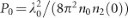. Then, we obtain: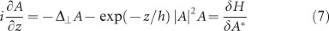where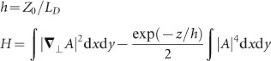For the parameters given above, we find LD=11 855 km, P0=0.339 GW and Pcr=4πP0=4.258 GW for a Gaussian input beam. The equation has a Hamiltonian structure.

The problem is characterized by two dimensionless parameters P/Pcr and h, where, typically, h<<1. One more dimensionless parameter related to the beam focusing will be introduced later.

There are several important and well-known relations related to Equation (7):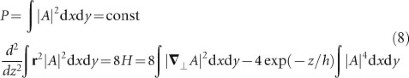Relation (8)—‘the Talanov theorem’7—is used to control numerical calculations. Usually, relation (8) is derived for uniform media, but it is also valid for the inhomogeneous case.

Let us consider the propagation of the initially Gaussian beam. On the surface, at z=0, we have: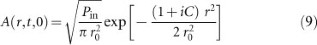where r0 is the initial beam size,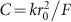is the initial beam prefocusing parameter (F represents a focal distance, which, in this case, is the debris height L) and Pin is the input power of the laser beam. C is the third dimensionless parameter of our problem, which is defined as C=LD/F. We solve the problem numerically for some specific parameters, but any situation with the same dimensional parameters will be equivalent. We numerically solve the Equation (7) in the domain 0≤zzm, 0≤rrm with zm=F=1000 km and rm/r0=10. At r=0, we use a symmetrical boundary condition, and at r=rm, the solution is set to A=0 or the solution of the linear problem.

We would like to stress that the problem under consideration, although similar to numerous other self-focusing studies in terms of the basic equation,8 is rather different in terms of the physics. The considered laser beam has a much larger spot size: over 1 m. The self-focusing length of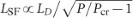is much longer than the thickness of the atmosphere. This moves the self-focusing (collapse) point far beyond the atmosphere. In other words, we consider here the propagation of light over a finite distance (the nonlinear layer of the finite thickness), and the collapse point is located beyond this region, where the propagation is linear. In this case, the self-focusing effect compresses the beam without the catastrophic collapse of all the energy into a small volume. This is a well-known nonlinear lens effect, and here, we can use it to relax the conditions on the size of the beam prefocusing mirrors. The numerical modeling strongly indicates that for the problem treated here, even for input powers well above the critical power for self-focusing, the beam can maintain its integrity and is compressed as a whole.

The calculations were performed for r0=1 m, L=1000 km and 1 μm light. The parameter C=Cmax for the optimal focusing in the linear case is 5.93. The distributions of the laser intensity in the focal plane for a few different values of P/Pcr are presented in Figure 2.

The intensities are normalized to the peak intensity for the linear case. One can observe a noticeable decrease in peak intensity for high P/Pcr. The effect increases with increasing power: I/Ilin is 0.8 for P/Pcr=760, 0.734 for P/Pcr=900, and 0.41 for P/Pcr=1500. The primary reason for this decrease is that the nonlinear lens focuses the radiation before the focal point of the corresponding linear problem. In Figure 3, we plot the intensity of the beam center as a function of z, for P/Pcr=1500, and we see that it peaks before the focal point, z=1000 km.

It is natural to attempt to compensate for the nonlinear effects with preliminary beam defocusing, in our case, by decreasing C. The results are presented in Figure 4. We see that proper initial defocusing can noticeably compensate the detrimental effects of nonlinearity.

The radial distribution of the beam intensity in the focal plane is presented in Figure 5.

We see that for the optimal defocusing, the peak intensity drops to only 0.7, in comparison with the non-compensated drop to 0.4; thus, the effect of self-focusing can be compensated for to a large extent.

Now, let us discuss the effect of the laser elevation. The nonlinear refractive index is proportional to the density of the air, so placing the laser at a high elevation is a natural method of reducing the detrimental nonlinear effects and the effects of propagation. We have already discussed the three dimensionless parameters used to characterize the problem: P/Pcr, LD/L and LD/Z0. From Equation (7), one can see that positioning the laser at a height h is equivalent to a decrease n2 by a factor of exp (−h/Z0) or an increase of Pcr by a factor of exp (h/Z0).

The height of the laser is small compared to the propagation distance L, and the change in LD/L can be disregarded. As a result, a change in the laser altitude is equivalent to a change in P/Pcr only. For example, the positioning of the laser at a height of 3 km reduces P/Pcr to 0.6 times its value at sea level. Positioning the laser at 4 km (the height of Mauna Kea) reduces this quantity to 0.51 times its sea-level value. Direct numerical modeling confirms these estimates; a change in the laser height is entirely equivalent to a reduction in P/Pcr.

A higher laser elevation is equivalent to a reduction in laser power compared to that of a sea-level laser. Therefore, the results presented in Figure 2 can be interpreted as an intensity distribution in the focal plane for a laser power of P/Pcr=1500 and laser elevations of 0, 3 and 4 km. We see that the laser elevation aids in decreasing the magnitude of the intensity reduction at the target. Initial defocusing helps to compensate for the drop in the same manner.

Let us qualitatively discuss the dependence of the self-focusing on various parameters. Consider the atmosphere as a nonlinear layer with a thickness of Z0=6 km. The beam modulation caused by nonlinear effects is characterized by the B integral,8,9 the nonlinear phase shift between the central and outer parts of a beam with radius a after propagation through the layer. B=1 or a phase difference of 2π is considered to be the boundary at which nonlinear effects become important. It is convenient to write the B integral in terms of laser power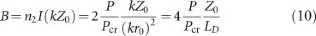For the above parameters and P/Pcr=1500, the B integral is approximately 3, and nonlinear effects are important. An increased laser elevation decreases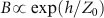and reduces the nonlinear effects.

Up to this point, all calculations have been performed for a fixed value of LD/L=10. Below, we present some calculations for a longer diffraction length and a mirror radius of r0=2 m. The B integral decreases by approximately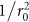, and the role played by nonlinear effects weakens rapidly. The radial distribution of intensity at the focal point is less affected by the self-focusing. The peak intensity for P/Pcr=1500 decreases by only 5% for linear focusing conditions and by only 3% for the optimal chirp. Further increasing the mirror radius to 3.5 m (7 m diameter) makes nonlinear effects negligible at this power. The peak power decreases by only 0.6%.

Further increasing the power eventually results in filamentation and rapid beam degradation. The filamentation begins in axisymmetric mode,10 and our treatment is adequate for the initial stage of the process.11 The destruction of the beam with increasing power is demonstrated in Figure 6. We observe a strong drop in peak intensity for P/Pcr>2000, with the formation of a ring filament, which is impossible to correct in a simple manner. Thus, to achieve reasonable focusing of the laser pulse, we must keep the B integral below 3–4.

Our results demonstrate that the intensity distribution for a situation without filamentation is close to Gaussian. In Figure 7, we present the phase at various heights as a function of r2. We see that the phase changes, but to a good approximation, it is proportional to r2. Thus, despite the nonlinear effects, the beam structure is locally close to a Gaussian shape, with beam size a and chirp C varying during the propagation: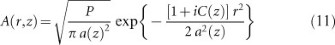This fact allows us to introduce a simplified description of the self-focusing. Using relation (8), one can obtain ordinary differential equations for a(z) and C(z). Analysis reveals that during the long propagation from the atmosphere to the focal point, even small deviations from the Gaussian shape are important, and the simplified description should be carefully adjusted to account for such deviations. The situation is different when a laser pulse propagates from orbit to the ground.6 In this case, the phase-front aberration in the atmosphere has no propagation distance over which to develop, and the propagation is less sensitive to atmospheric propagation effects.

Next, we discuss the key processes that affect beam propagation.

Atmospheric turbulence scatters light and causes broadening of the propagated beam. The scattering is induced by density and temperature perturbations, resulting in fluctuations of the refractive index. Let us estimate the effect of turbulence on the focusing of laser radiation.

Atmospheric turbulence is usually treated as isotropic and uniform, using the Kolmogorov spectrum of turbulence. In this situation, the correlation function for fluctuations in the refractive index n(r) satisfies the relation: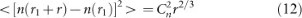The turbulence is characterized by the constant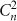. Typical values of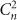are in the range of 10−13–10−15 m−2/3 near the ground and decrease with increasing height.

The most common semiempirical model used to describe the beam broadening caused by turbulence in the linear approximation uses the following expression for the beam radius on the target rt: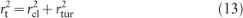where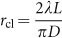is the diffraction-limited spot radius, L is the height of the debris orbit and D is the diameter of the focusing mirror. In practical situations, spot size often must be increased by the beam quality factor.

For turbulent broadening, we use the Dowling–Breaux model,12 which is based on both theoretical studies and experimental results. The most important parameter in the model is the Fried coherence length r0: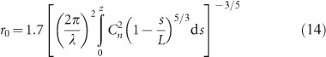In our case of a thin atmosphere, this expression can be rewritten as: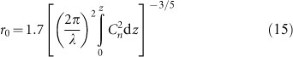In terms of r0, the spot radius at the target can be represented as:12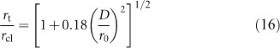In Equation (14), the focusing mirror is located at z=0, and the focal plane is at z=L. The factor (1−s/L) in Equation (14) indicates that scattering near the focus is less important than scattering near the mirror; a ray scattered near the mirror deviates from the beam axis even for free propagation, whereas a ray scattered near the focal spot has no time to deviate. This effect is unimportant in our case.

To proceed, we need a model of atmospheric turbulence. One simple model that is used frequently assumes that the turbulence is maximal near the surface and, beginning at a height of z=z0=10 m, decreases proportionally to 1/z. Consider the height dependence as follows: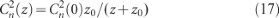This model is applicable up to an altitude of approximately 3 km; beyond that, a more complicated model with an exponential decline in Cn, i.e., the Hafnagel model,12 must be used. For simplicity, we set Cn equal to zero at the height h=6 km. For low turbulence levels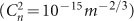, the Fried coherence length will be approximately 1 m, and even for a mirror with a diameter of 2 m, turbulence can substantially broaden the beam. The turbulence effect can be greatly reduced by placing the laser on a high mountain, but even in this case, it can be problematic for a large-diameter mirror.

The most important nonlinear process that can affect powerful beam propagation is stimulated Raman scattering. The dominant Raman process is rotational Raman scattering by nitrogen (SRRS).13,14 In the atmosphere, pressure broadening dominates up to a height of L approximately 40 km, and the gain coefficient is independent of density and does not change substantially:13,14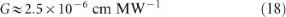The total gain necessary for Raman scattering to grow from the level of noise to a level that is high enough to destroy the beam is gIL≈20. From this value, we find that the SRRS is appreciable for intensities of 2 MW cm−2.

The above estimate assumes stationary SRRS. For pulses shorter than the Raman relaxation time, SRRS is in the non-stationary regime, and the process threshold is increased.13,14 The relaxation time changes from 0.1 ns on the ground to 10 ns at 40 km. The threshold intensity is approximately 10 MW cm−2 for a 1 ns pulse and approximately 100 MW cm−2 for a 0.1 ns pulse. Thus, by using shorter pulses, we can suppress Raman scattering.

A higher laser elevation reduces the amplification length only slightly (approximately 10% for h≈4 km), but increasing the relaxation time can greatly increase the Raman threshold.

We should mention that the above estimates of the effects of Raman scattering are conservative. The threshold calculation14 assumes that 1% of the radiation is converted into scattered light. Raman scattering peaks in the forward direction, and energy losses are minimal. Even noticeable scattering may not affect the irradiation of the target.

We note that the suppression of the various detrimental effects implies contradictory requirements. To suppress Raman scattering, we must increase the director diameter (to reduce the intensity). Doing so also reduces the self-focusing and most importantly, according to Equation (5), the required laser pulse energy, which makes systems with large mirrors attractive prospects.2 However, large-diameter mirrors also enhance the beam broadening caused by atmospheric turbulence, making beam control more difficult.

Shortening the pulse to suppress Raman scattering decreases the efficiency of the laser system and increases the self-focusing. The design of a laser system for debris clearing must optimize both the physics and engineering requirements. Nonetheless, one thing is clear: a laser elevation of approximately 4 km will greatly improve the system performance.

## Conclusions

We demonstrated that for a ground-based laser space-debris cleaning system, self-focusing could greatly affect the beam propagation. Because of the finite thickness of the atmosphere, the self-focusing does not filament the beam and changes only its macroscopic parameters: focal length and beam size. We showed that initial beam defocusing can, to a large extent, compensate for the detrimental effects of nonlinearity.